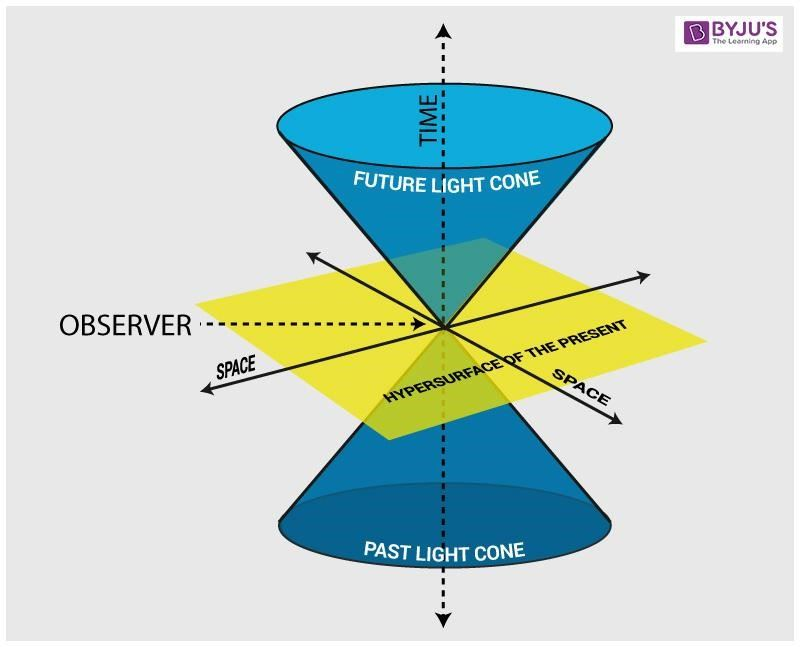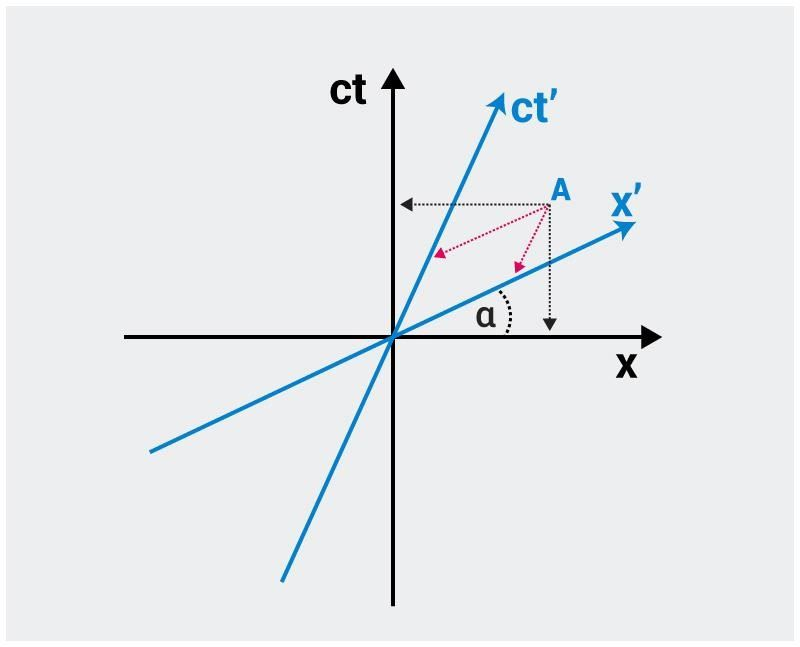# Minkowski Space

## What Is Minkowski Space?

Minkowski space or Minkowski Spacetime terms are used in mathematical physics and special relativity. It is basically a combination of 3-dimensional Euclidean Space and time into a 4-dimensional manifold, where the interval of spacetime that exists between any two events is not dependent on the inertial frame of reference. This concept was initially developed by the Scientist Hermann Minkowski for Maxwell’s equation of electromagnetism.The mathematical structure of Minkowski spacetime was an immediate consequence of the postulates of relativity. The individual components in Euclidean space and time vary due to time dilation and length contraction. All the reference frames in Minkowski spacetime agrees on the overall distance in the spacetime between the events, this is because it treats the 4th dimension (time) differently than the 3 spatial dimensions. The metric signature of Minkowski spacetime is represented as (-+++) or (+—) and it is always flat.

### Mathematical Definition

Minkowski spacetime is a 4-dimensional coordinate system in which the axes are given by (x, y, z, ct)

We can also write as ( $$x_{1},x_{2},x_{3},x_{4}$$ )

Here we have written ct as $$x_{4}$$, here time is measured in units of speed of light times the time coordinates this is because the unit of time should be same as the unit for space.

The differential for arc length in spacetime is given by the equation:

 $$ds^{2}=-c^{2}dt^{2}+dx^{2}+dy^{2}+dz^{2}$$

This equation has a metric tensor of spacetime given by:

$$g_{uv}=\begin{bmatrix} -1 & 0 & 0 & 0\\ 0 & 1 & 0 & 0\\ 0 & 0 & 1 & 0\\ 0 & 0 & 0 & 1 \end{bmatrix}$$

As stated before, spacetime is a flat everywhere.

### Minkowski Diagrams

The scientist used a separate graphing system called Minkowski diagrams to properly demonstrate the properties of Lorentz transformation and relate the Newtonian mechanics to relativistic mechanics using proper graphical methods.As you see in the image different coordinate systems will not agree on the object’s position or spatial orientation in time. We can see that there is 1 spatial axis i.e. x-axis and the other is the time axis i.e. ct-axis.

The Minkowski Spacetime has a different set of rules for graphing which are as follows:

1. $$tan\Theta =\frac{v}{c}$$ is the angle between the x-axis and x`-axis where ‘v’ is the object’s velocity.
2. The speed of light in the spacetime always makes an angle of 45° with either axis.

 $$R_{uv}-\frac{1}{2}g_{uv}R=8\Pi T_{uv}$$